## [原创] 用人话解释机器学习中的Logistic Regression（逻辑回归）

Logistic Regression（或Logit Regression），即逻辑回归，简记为LR，是机器学习领域的一种极为常用的算法／方法／模型。

## [原创] 再谈 梯度下降法/最速下降法/Gradient descent/Steepest Descent

『1』名字释义

『2』函数值下降最快的方向是什么

$f(x) = f({x_k}) + \alpha g_k^T{d_k} + o(\alpha )$

$({a_1}{b_1} + {a_2}{b_2} + \cdots + {a_n}{b_n}) \le \sqrt {(a_1^2 + a_2^2 + \cdots + a_n^2)} \sqrt {(b_1^2 + b_2^2 + \cdots + b_n^2)}$

$\frac{{{a_1}}}{{{b_1}}} = \frac{{{a_2}}}{{{b_2}}} = \cdots = \frac{{{a_n}}}{{{b_n}}}$

$\left| {d_k^T{g_k}} \right| \le \left\| {{d_k}} \right\|\left\| {{g_k}} \right\|$

『3』缺点

『4』感受一下它是如何“慢”的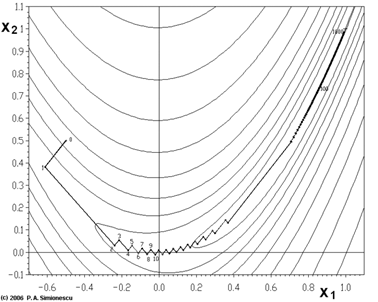$f({x_1},{x_2}) = {(1 - {x_1})^2} + 100 \cdot {({x_2} - {x_1}^2)^2}$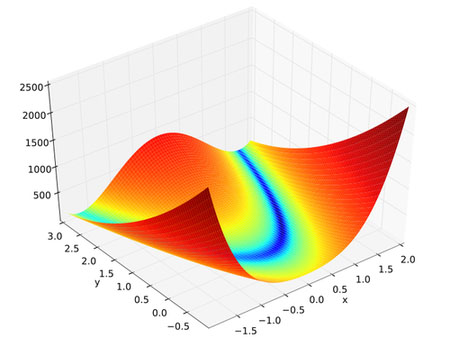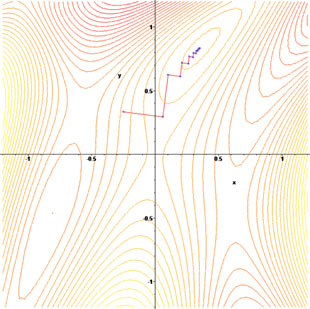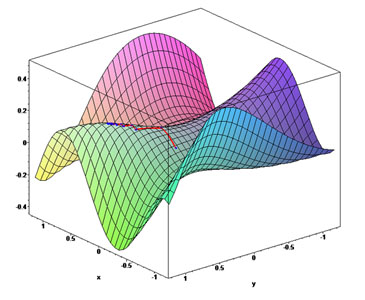『5』为什么“慢”

$\nabla f{({x_k} + {\alpha _k}{d_k})^T}{d_k} = 0$ ，即 $g_{k + 1}^T{d_k} = 0$

$g_{k + 1}^T{d_k} = g_{k + 1}^T( - {g_k}) = - g_{k + 1}^T{g_k} = - d_{k + 1}^T{d_k} = 0 \Rightarrow$ $d_{k + 1}^T{d_k} = 0$

$\cos \theta = \frac{{{d_k}^T{d_k}}}{{\left\| {{d_k}} \right\|\left\| {{d_k}} \right\|}} = \frac{0}{{\left\| {{d_k}} \right\|\left\| {{d_k}} \right\|}} = 0\;$
=> $\theta = \frac{\pi }{2}$

『6』优点

『7』收敛性及收敛速度

• 引用
（1）https://en.wikipedia.org/wiki/Cauchy%E2%80%93Schwarz_inequality
（3）https://en.wikipedia.org/wiki/Rosenbrock_function

## [原创] Cauchy-Schwartz(柯西-许瓦兹)不等式复习

${({a_1}{b_1} + {a_2}{b_2} + \cdots + {a_n}{b_n})^2} \le (a_1^2 + a_2^2 + \cdots + a_n^2)(b_1^2 + b_2^2 + \cdots + b_n^2)$

## [原创] line search中的重要定理 - 梯度与方向的点积为零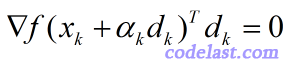## [原创]用“人话”解释不精确线搜索中的Armijo-Goldstein准则及Wolfe-Powell准则

line search（一维搜索，或线搜索）是最优化（Optimization）算法中的一个基础步骤/算法。它可以分为精确的一维搜索以及不精确的一维搜索两大类。